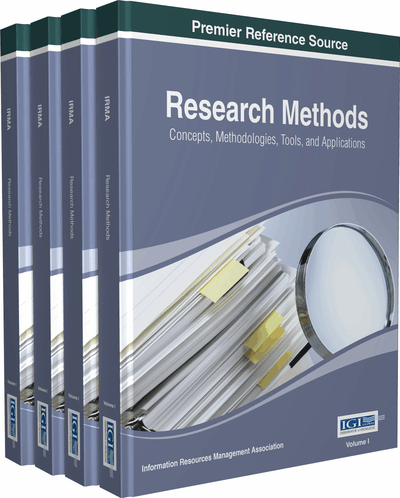# Demonic Fuzzy Relational Calculus

Fairouz Tchier (King Saud University, Saudi Arabia) and Huda Alrashidi (King Saud University, Saudi Arabia)
DOI: 10.4018/978-1-4666-7456-1.ch018

## Abstract

In this chapter, the authors categorize methods that are used to formally specify and verify software requirements. They discuss several formal method-related subjects such as calculus fuzzy and relational calculus.
Chapter Preview
Top

## Motivation

The importance of relations is almost self-evident. Science is, in a sense, the discovery of relations between observables. Zadeh has shown the study of relations to be equivalent to the general study of systems (a system is a relation between an input space and an output space.) (Goguen, 2009).

The calculus of relations has been an important component of the development of logic and algebra since the middle of the nineteenth century. George Boole, in his “Mathematical Analysis of Logic” (Boole, 1847), initiated the treatment of logic as part of mathematics, specifically as part of algebra. Quite the opposite conviction was put forward early this century by Bertrand Russell and Alfred North Whitehead in their Principia Mathematica (Whitehead & Russell, 1910): that mathematics was essentially grounded in logic. The logic is developed in two streams. On the one hand algebraic logic, in which the calculus of relations played a particularly prominent part, was taken up from Boole by Charles Sanders Peirce, who wished to do for the “calculus of relatives” what Boole had done for the calculus of sets, Peirce’s work was in turn taken up by Schröder in “Algebra und Logik der Relative.” Schröder’s work, however, lay dormant for more than 40 years, until revived by Alfred Tarski in his seminal paper “On the Calculus of Binary Relations” (Tarski, 1941). Tarski’s paper is still often referred to as the best introduction to the calculus of relations. It gave rise to a whole field of study, that of relation algebras, and more generally Boolean algebras with operators. This important work defined much of the subsequent development of logic in the 20th century, completely eclipsing for some time the development of algebraic logic. In this stream of development, relational calculus and relational methods appear with the development of universal algebra in the 1930’s, and again with model theory from the 1950s onwards. In so far as these disciplines in turn overlapped with the development of category theory, relational methods sometimes appear in this context as well. It is fair to say that the role of the calculus of relations in the interaction between algebra and logic is by now well understood and appreciated, and that relational methods are part of the toolbox of the mathematician and the logician.

The main advantages of the relational formalization are uniformity and modularity. Actually, once problems in these fields are formalized in terms of relational calculus, these problems can be considered by using formulae of relations, that is, we need only calculus of relations in order to solve the problems. This makes investigation on these fields easier.

Over the past twenty years relational methods have however also become of fundamental importance in computer science. For example, much of the theory of nonclassical logics is used (though sometimes re-invented) in the new so-called program logics. These arose from the realization that a program may be thought as an input-output relation over some state space: an accessibility relation. This point of view, that the calculus of relations is fundamental to programs, was clearly enunciated by the Oxford Group of Tony Hoare in an influential paper on “Laws of Programming” (Hoare, et al., 1987). Also during the 1980s, much of the equational theory of relation algebras were already being applied to program semantics and program development. For example, the book Relations and Graphs by Schmidt and Strohlein (in German, 1989 [Schmidt & Ströhlein, 1989]; in English, 1993 [Schmidt & Ströhlein, 1993]) started from the basis of programs as graphs with Boolean matrices. Thus computer science, as the new application field for relational methods, has both drawn from and contributed to previous logic/mathematical work; this is a sign of healthy development.

## Complete Chapter List

Search this Book:
Reset